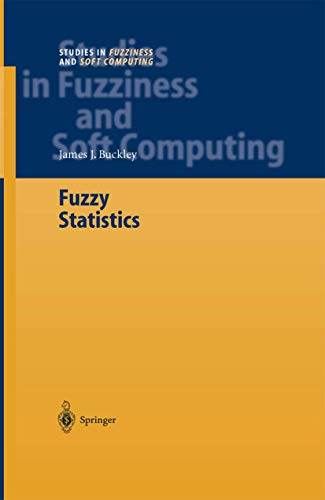• Free Shipping on all orders in Australia
• Over 7 million books in stock
• Proud to be B-Corp
• We aim to be carbon neutral by 2022
• Over 120,000 Trustpilot reviews
Item 1 of 0# Fuzzy Statistics by James J. Buckley

Condition - New
\$227.09
Only 2 left

## Summary

For example, in Chapter 12 we consider the test Ho : /1 = /10 verses HI : /1 f=- /10 where /1 is the mean of a normal distribution with known variance, but we use a fuzzy number (from Chapter 3) estimator of /1 in the test statistic. Part four, Chapters 21-27, are on fuzzy regression and fuzzy prediction.

This book will be specially printed for your order, which means it may take a little longer to arrive (5 days). We do this to cut down on waste & to help protect our planet.

## Fuzzy Statistics Summary

### Fuzzy Statistics by James J. Buckley

1. 1 Introduction This book is written in four major divisions. The first part is the introductory chapters consisting of Chapters 1 and 2. In part two, Chapters 3-11, we develop fuzzy estimation. For example, in Chapter 3 we construct a fuzzy estimator for the mean of a normal distribution assuming the variance is known. More details on fuzzy estimation are in Chapter 3 and then after Chapter 3, Chapters 4-11 can be read independently. Part three, Chapters 12- 20, are on fuzzy hypothesis testing. For example, in Chapter 12 we consider the test Ho : /1 = /10 verses HI : /1 f=- /10 where /1 is the mean of a normal distribution with known variance, but we use a fuzzy number (from Chapter 3) estimator of /1 in the test statistic. More details on fuzzy hypothesis testing are in Chapter 12 and then after Chapter 12 Chapters 13-20 may be read independently. Part four, Chapters 21-27, are on fuzzy regression and fuzzy prediction. We start with fuzzy correlation in Chapter 21. Simple linear regression is the topic in Chapters 22-24 and Chapters 25-27 concentrate on multiple linear regression. Part two (fuzzy estimation) is used in Chapters 22 and 25; and part 3 (fuzzy hypothesis testing) is employed in Chapters 24 and 27. Fuzzy prediction is contained in Chapters 23 and 26. A most important part of our models in fuzzy statistics is that we always start with a random sample producing crisp (non-fuzzy) data.

## Table of Contents

Fuzzy Sets.- Estimate ?, Variance Known.- Estimate ?, Variance Unknown.- Estimate p, Binomial Population.- Estimate ?2 from a Normal Population.- Estimate 1 - 2, Variances Known.- Estimate ? 1 - ? 2, Variances Unknown.- Estimate d =? 1 - ? 2, Matched Pairs.- Estimate p 1 - p 2, Binomial Populations.- Estimate ? 1 2 /? 2 2 , Normal Populations.- Tests on , Variance Known.- Tests on , Variance Unknown.- Tests on p for a Binomial Population.- Tests on ? 2, Normal Population.- Tests ? 1 verses ? 2, Variances Known.- Test ? 1 verses ? 2, Variances Unknown.- Test p 1 = p 2, Binomial Populations.- Test d = 1 - 2 , Matched Pairs.- Test ? 1 2 verses ? 2 2 , Normal Populations.- Fuzzy Correlation.- Estimation in Simple Linear Regression.- Fuzzy Prediction in Linear Regression.- Hypothesis Testing in Regression.- Estimation in Multiple Regression.- Fuzzy Prediction in Regression.- Hypothesis Testing in Regression.- Summary and Questions.- Maple Commands.

## Additional information

NLS9783642059247
9783642059247
3642059244
Fuzzy Statistics by James J. Buckley
New
Paperback
Springer-Verlag Berlin and Heidelberg GmbH & Co. KG
2010-12-15
168
N/A
Book picture is for illustrative purposes only, actual binding, cover or edition may vary.
This is a new book - be the first to read this copy. With untouched pages and a perfect binding, your brand new copy is ready to be opened for the first time